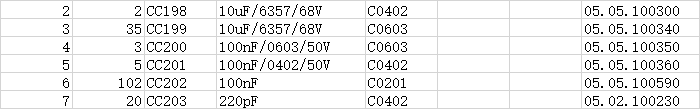# Excel 多表数据模糊查询匹配，并提取对应数据到原始表2021 年 06 月 30 日08:54:13

```import openpyxl

epath = r"C:\Users\xuehu\Desktop\1.xlsx"
epath_va = r"C:\Users\xuehu\Desktop\2.xlsx"

# 一些配置信息
# 第一个数据表的数据起始行
first_excel_from = 10
# 第一个数据表格中需要匹配的数据列
first_excel_data_one = 4
# 第一个数据表中需要匹配的数据列
first_excel_data_two = 5
# 第二个数据表的起始行
second_excel_data = 1
# 第二个数据表中检测匹配的数据列
second_excel_checkdata = 6
# 第二个数据表中需要提取的数据列
second_excel_getdata = 5

tnum = 0

booksheet = workbook.active
rows = booksheet.rows
columns = booksheet.columns
nowrow = 0

workbook_w = openpyxl.Workbook()
workbook_w.save("DATA.xlsx")
sheet = workbook_w.active

for row in rows:
nowrow = nowrow + 1
nowcell = 0
# 开始读取行数据
if nowrow > first_excel_from:
# 开始读取列数据
# 定义列数据表
orgdata = []
newdata = []
for cell in row:
newdata.append(cell.value)
nowcell = nowcell + 1
if nowcell == first_excel_data_one or nowcell == first_excel_data_two:
# 将关键的匹配信息取出来
orgdata.append(cell.value)
# print(cell.value, end=',')
# print(orgdata)
# 读取第二个匹配表的数据，对比，并提取数据
va_booksheet = va_workbook.active
va_rows = va_booksheet.rows
va_columns = va_booksheet.columns
va_rownum = 0
for va_row in va_rows:
va_rownum = va_rownum + 1
va_cellnum = 0
if va_rownum > second_excel_data:
for va_cell in va_row:
if va_cell.value is None:
va_cell.value = " "
va_cellnum = va_cellnum + 1
if va_cellnum == second_excel_checkdata:
# print(va_cell.value)
# 执行数据匹配
tnum = 0
for st in orgdata:
if st in va_cell.value:
tnum = tnum + 1
if va_cellnum == second_excel_getdata:
if tnum == 2:
newdata.append(str(va_cell.value))
# print(va_cell.value)
# print("============第二个表行结束================")
print(newdata)
sheet.append(newdata)
# print("===========行结束===========")
workbook_w.save("DATA.xlsx")```##### 历史上的今天
6月
30
• 免费优惠券
• 免费领取优惠券
•• 品质与测试
• 关注品质与测试
•• 版权声明：本文为原创文章，转载请附上原文出处链接及本声明。
• 转载请注明：Excel 多表数据模糊查询匹配，并提取对应数据到原始表 | https://chenxuehu.com/article/2021/06/7743.html